# 金融数据获取的api接口

+关注继续查看

import pandas_datareader.data  as web
import datetime
import matplotlib.pyplot as plt
from matplotlib.pylab import date2num
from matplotlib.font_manager
import FontProperties
import mpl_finance as mpf
import matplotlib
import numpy as np
matplotlib.style.use('ggplot')
%matplotlib inline


start = datetime.datetime(1980, 1,1)
end = datetime.datetime(2018,8,3)
prices = web.get_data_yahoo('AAPL',start, end)

#预览股价趋势图
prices['Close'].plot()
plt.show()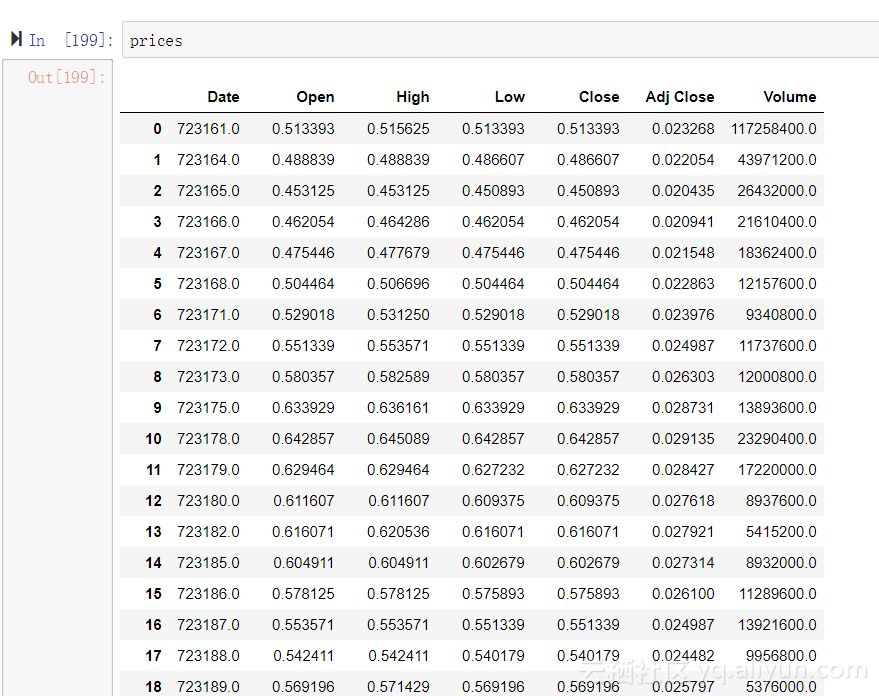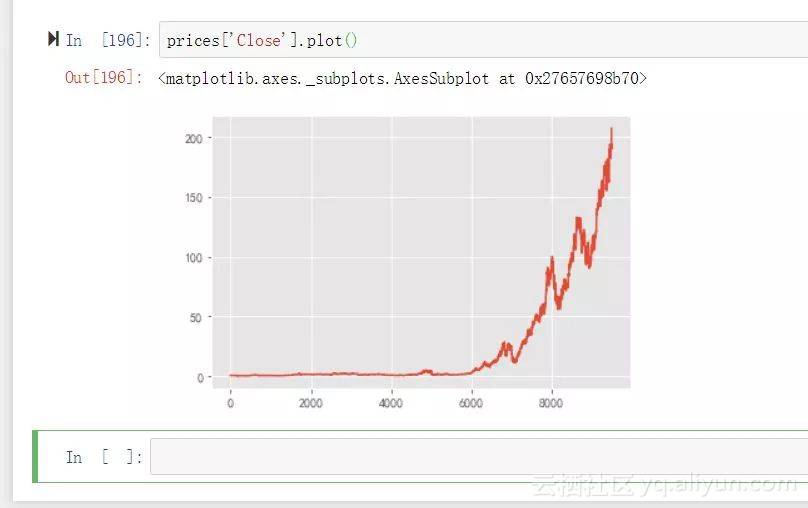def create_candles(data):
fig, ax = plt.subplots(figsize=(16,10))
ax.xaxis_date()
plt.xticks(rotation=45)
plt.yticks()
plt.rcParams['font.sans-serif']=['SimHei']
plt.title("股票代码：APPL苹果历史股价")
plt.xlabel("时间")
plt.ylabel("股价（元）")
mpf.candlestick_ohlc(ax,data,width=1.5,colorup='r',colordown='green')
plt.grid(True)


if __name__ == '__main__':
prices = prices.reset_index()
prices['Date'] = prices['Date'].map(lambda x: date2num(x))
data = prices.iloc[:,:5].values
create_candles(data)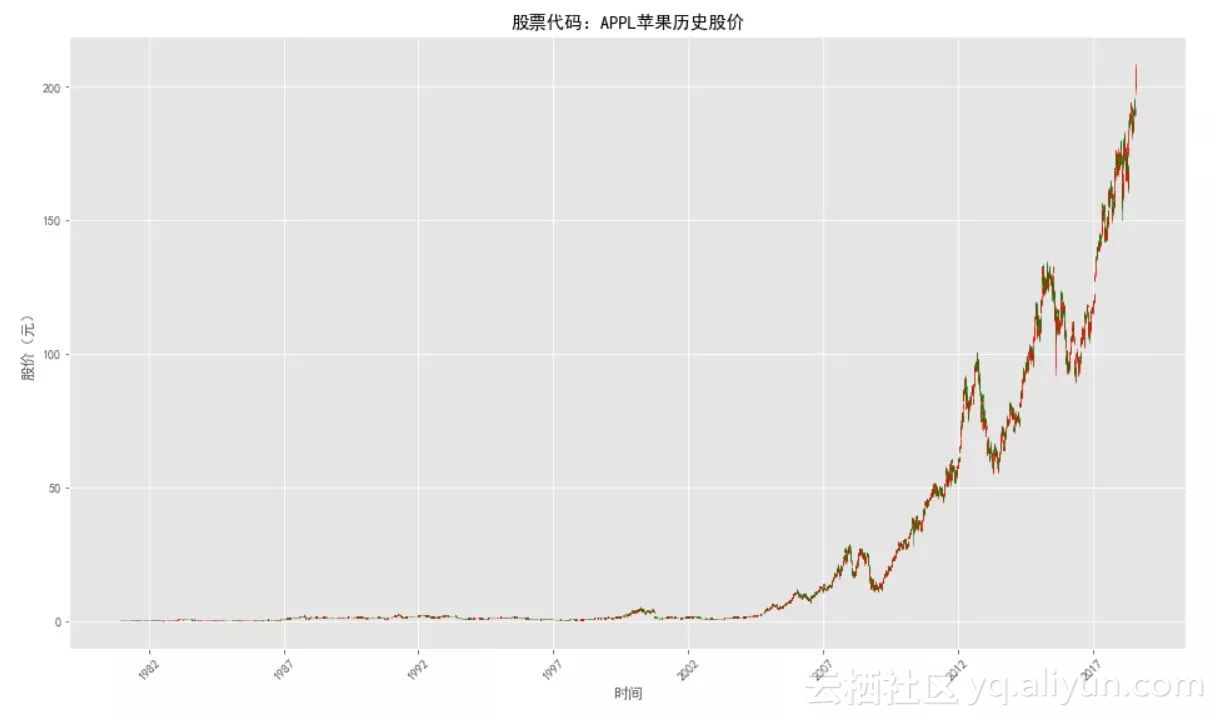tushare包是一个非常优秀的金融信息数据接口包，虽然取法覆盖港股和美股，但是对于国内的沪深股市数据支持的非常完善。

ts.get_k_data('000001', index=True)                #交易数据：
ts.get_hist_data('600848')                         #一次性获取全部日k线数据
ts.get_h_data()                                    #获取历史复权数据
ts.get_today_all()                                 #实时行情
df = ts.get_tick_data('600848',date='2014-01-09')  #历史分笔交易


R语言中支持金融数据获取的接口自然要数quantmood包了。

library("quantmod")
#指定要获取的股票名称或代码，以及数据源
setSymbolLookup(JJKR=list(name="AAPL",src="yahoo"))
getSymbols("AAPL")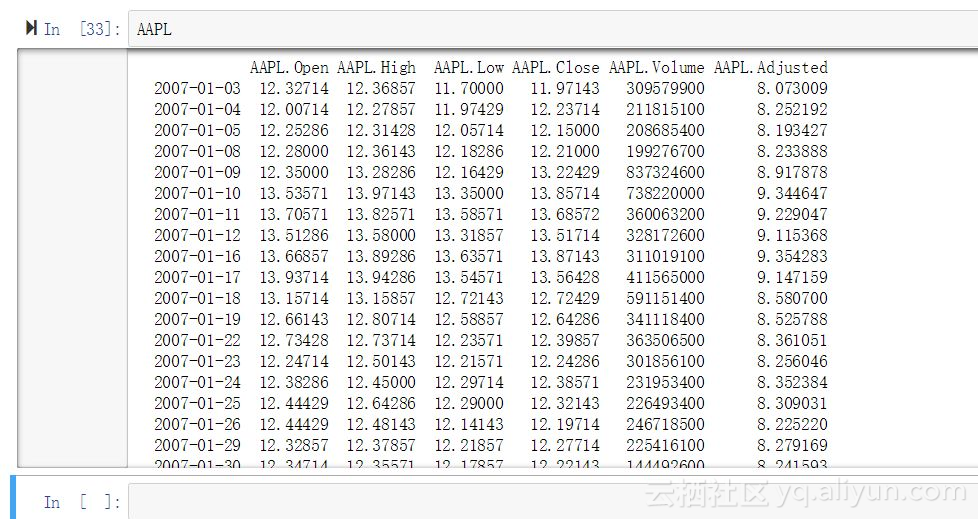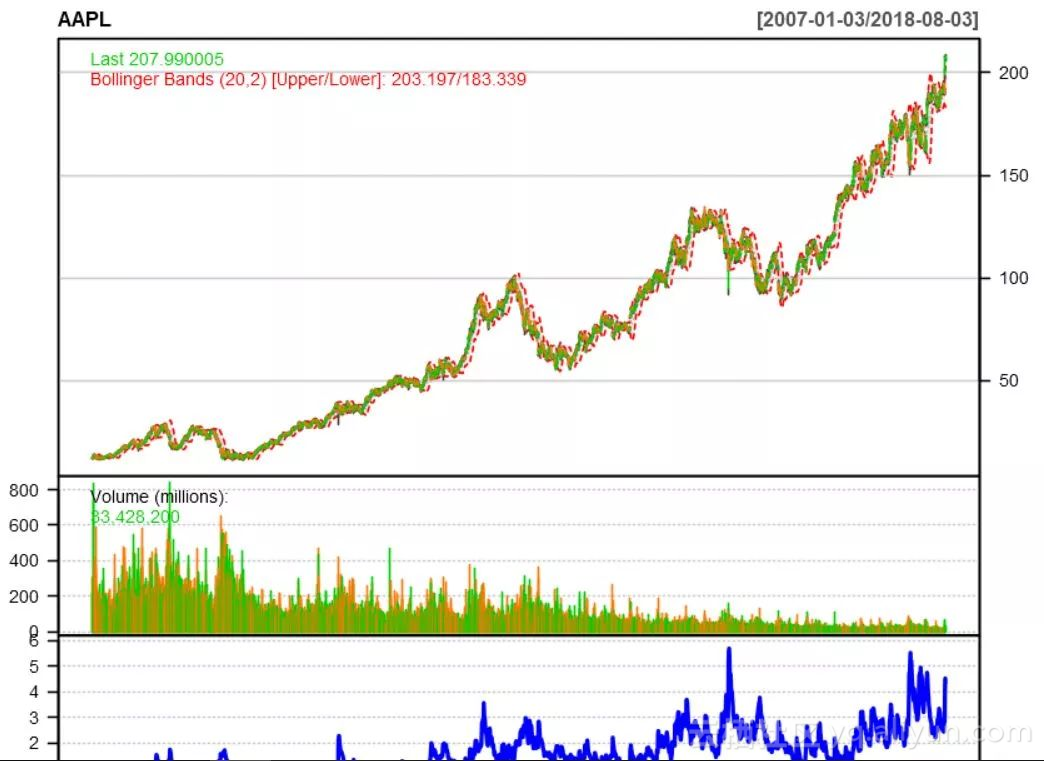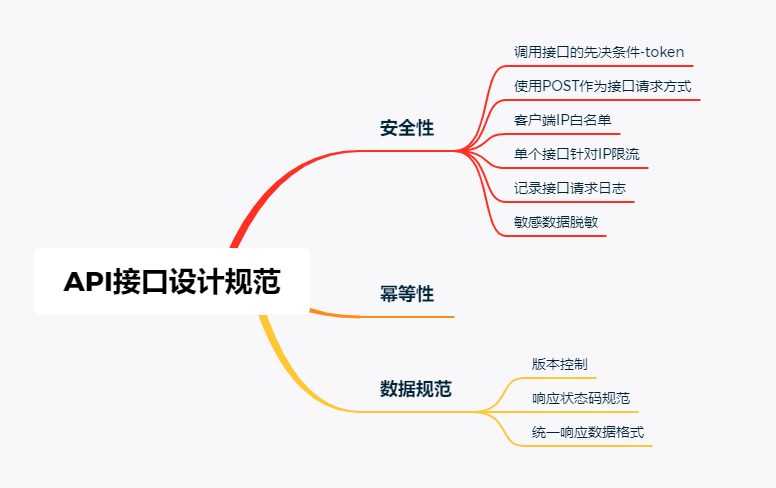0 0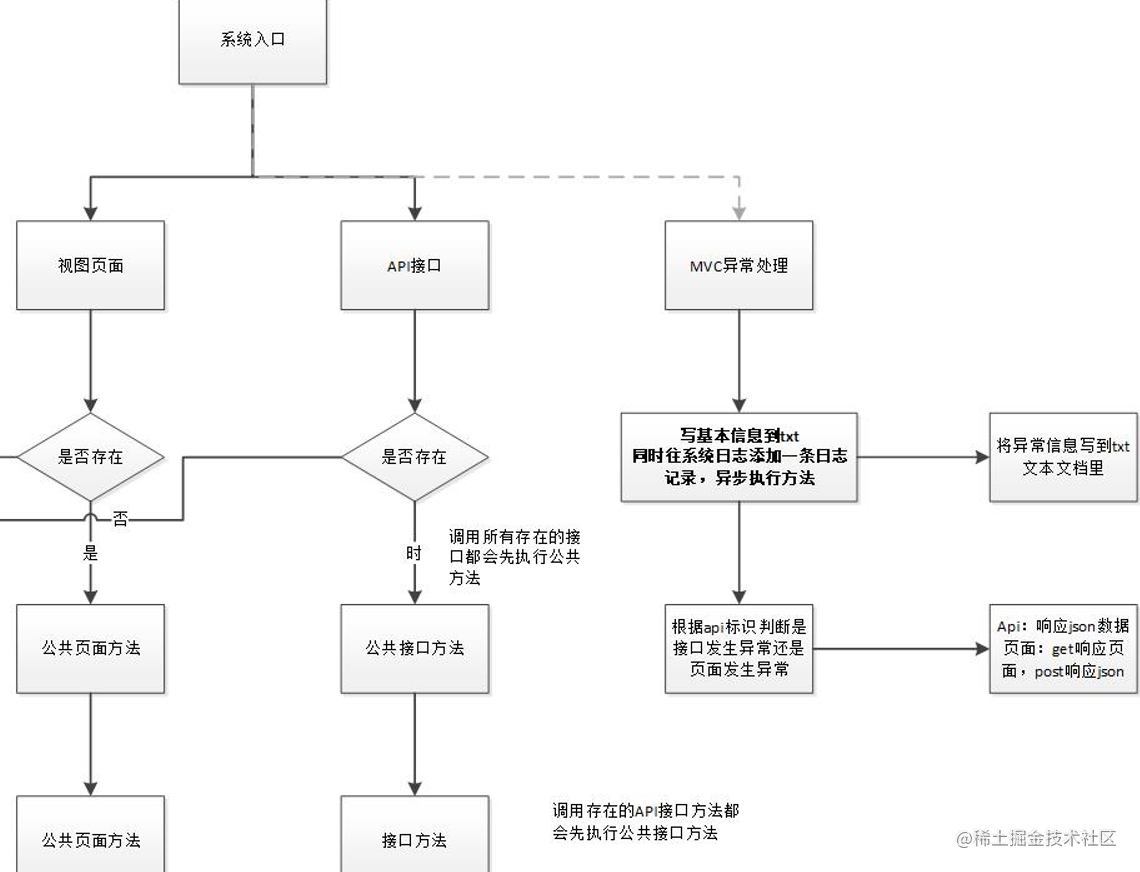【C#】.net core2.1，自定义全局类对API接口和视图页面产生的异常统一处理

0 0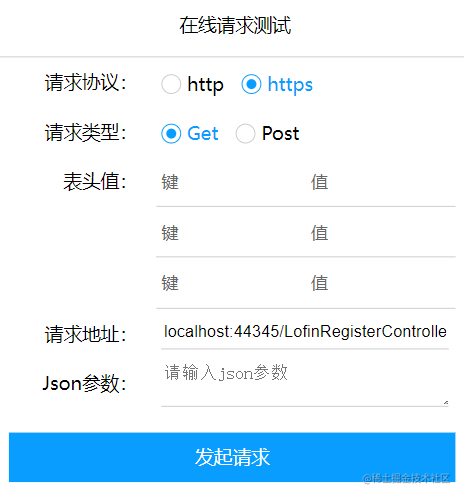【前端】使用jQuery实现一个简单的在线页面或API接口请求功能

0 0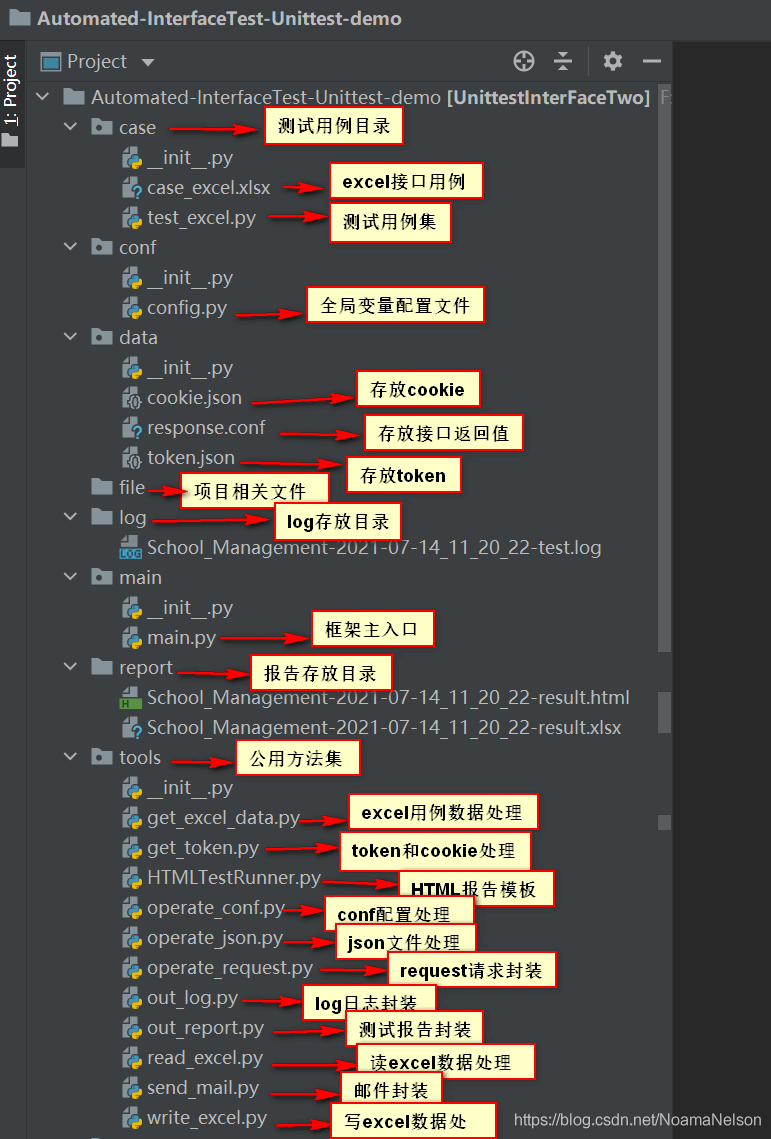API接口自动化测试框架搭建之需求整理、详细设计和框架设计
API接口自动化测试框架搭建之需求整理、详细设计和框架设计
0 0h5 的api接口 地理位置,重力感应,摇一摇,requestAnimationFrame,localStorage,history,worker
h5 的api接口 地理位置,重力感应,摇一摇,requestAnimationFrame,localStorage,history,worker
0 00 00 0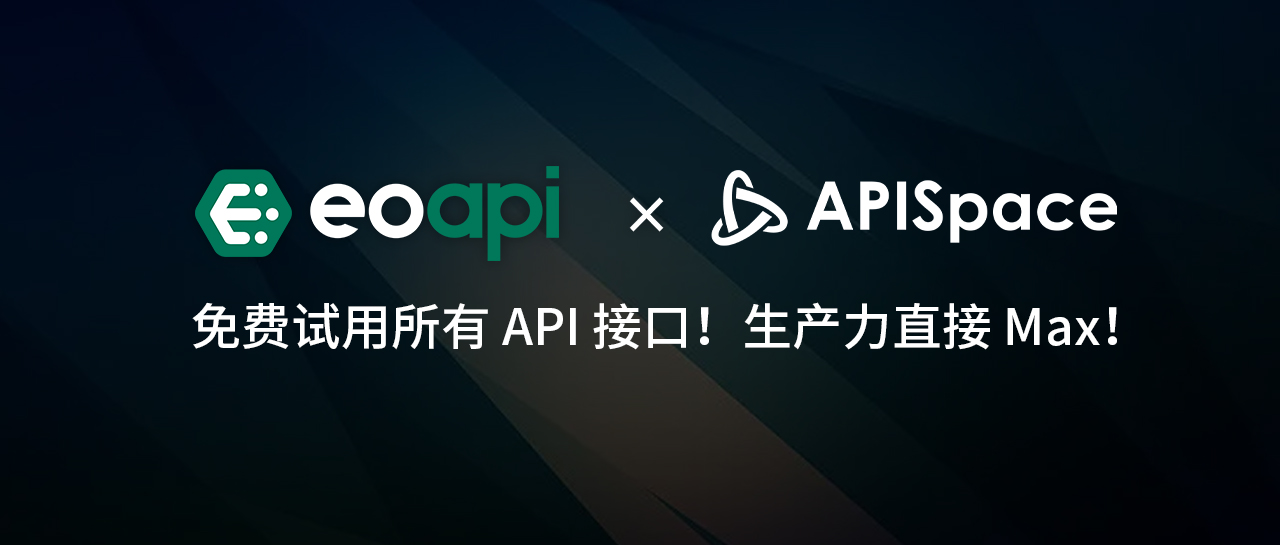Eoapi x APISpace：免费试用所有 API 接口！生产力直接 Max！

0 0138727941643514432

CUDA Math API